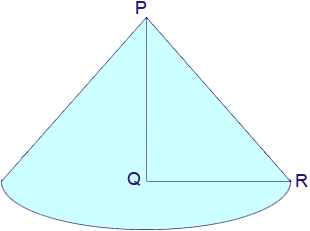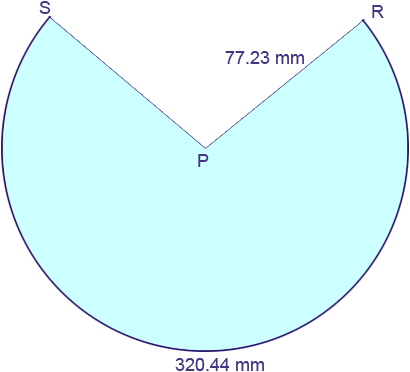Hi there, I am an art student and in the process of making a cone out of sheet metal. I am unable to work out the template I need to produce my final cone. The dimensions I have are that the final cone will be 58mm high and will have a diameter of 102mm. Can you help? Thanks, Laura Hi Laura, I drew a picture of your cone and labeled some points.|PQ| = 58 mm and |QR| = 51 mm and the triangle PQR is a right triangle so be Pythagoras Theorem |PR|2 = |PQ|2 + ||QR|2 = 582 + 512 = 3364 + 2601 = 5965 so |PR| = √5965 = 77.23 mm. Thus when you cone is rolled out flat it is a sector of a circle of radius 77.23 mm.The length of the arc RS is the circumference of the circle at the base of your cone. This is a circle of radius 51 mm and the circumference of a circle of radius r is given by circumference = 2r = 251 = 320.44 mm. All that remains is to determine the measure of the angle RPS. The relationship between the length of the arc of a sector of a circle, the radius of the circle and the angle at the center is arc length = radiusangle where the angle is measured in radians. Thus 320.44 = 77.23angle so angle = 320.44/77.23 = 4.15 radians. There areradians in 180o and hence 4.15 radians is 4.15180/= 237.7o Hence you need to draw a circle of radius 77.23 mm, cut out a sector with centre angle 360o - 237.7o = 122.3o . What remains is your template. Penny